Standardized Solutions

Standardized solutions are useful when you want to compare parameter values that are measured on quite different scales. PROC CALIS provides standardized solutions routinely. In standardizing a solution, parameters are classified into five groups:

• path coefficients, regression coefficients, or direct effects
With each parameter
in this group, there is an associated outcome variable and a predictor variable. Denote the predicted variance of the outcome variable by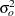and the variance of the predictor variable by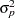, the standardized parameteris: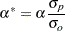• fixed ones for the path coefficients attached to error or disturbance terms
These fixed values are unchanged in standardization.

• variances and covariances among exogenous variables, excluding errors and disturbances
Let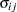be the covariance between variablesand. In this notation,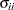is the variance of variable. The standardized covariance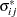is: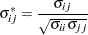When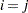,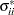takes the value offor all. Also,is the correlation between the-th and-th variables.

• variances and covariances among errors or disturbances
Denote the error covariance parameter as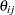so that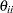represents the variance parameter of error variable. Associated with each error or disturbance variableis a unique outcome variable. Let the variance of such an outcome variable be. In the standardized solution, the error covarianceis rescaled as: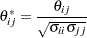Notice that when,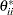is not standardized toin general. In fact, the error (disturbance) variance is simply rescaled by the reciprocal of the variance of the associated dependent variable. As a result, the rescaled error (disturbance) variance represents the proportion of variation of the dependent variable due to the unsystematic source. By the same token,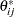does not represent the correlation between errorsand. It is a rescaled covariance of the errors involved.

• intercepts and means of variables
These parameters are fixed zeros in the standardized solution.

While formulas for the standardized solution are useful in computing the parameter values in the standardized solution, it is conceptually more useful to explain how variables are being transformed in the standardization process. The following provides a summary of the transformation process:

• Observed and latent variables, excluding errors or disturbances, are centered and then divided by their corresponding standard deviations. Therefore, in the standardized solution, all these variables will have variance equal to. In other words, these variables are truly standardized.

• Errors or disturbances are divided by the standard deviations of the corresponding outcome variables. In the standardized solution, these variables will not have variance equal toin general. However, the rescaled error variances represent the proportion of unexplained or unsystematic variance of the corresponding outcome variables. Therefore, errors or disturbances in the standardized solution are simply rescaled but not standardized.

Standardized total, direct, and indirect effects are computed using formulas presented in the section Total, Direct, and Indirect Effects, but with the standardized parameter values substituted into the formulas.

Although parameter values associated with different scales are made more comparable in the standardized solution, a precaution should be mentioned. In the standardized solution, the original constraints on parameters in the unstandardized solution are usually lost. These constraints, however, might underscore some important theoretical position that needs to be maintained in the model. Destroying these constraints in the standardized solution means that interpretations or comparisons of parameter values in the standardized solution are made without maintaining the original theoretical position. You must judge whether such a departure from the original constraints poses conceptual difficulties for interpreting the standardized solution.